# Inner product

(diff) ← Older revision | Latest revision (diff) | Newer revision → (diff)

scalar product, dot product,of two non-zero vectors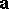andThe product of their lengths and the cosine of the anglebetween them:is taken to be that angle between the vectors not exceeding. Whenoris zero, the inner product is taken to be zero. The inner productis called the scalar square of the vector(see Vector algebra).

The inner product of two-dimensional vectors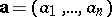and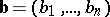over the real numbers is given byIn the complex case it is given by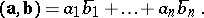An infinite-dimensional vector space admitting an inner product and complete with respect to it is called a Hilbert space.

More generally, an inner product on a real vector space is a symmetric bilinear formwhich is positive definite, i.e.,for all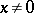. A (unitary) inner product on a complex vector space is likewise defined as a Hermitian (i.e., with) sesquilinear form, with complex conjugation as automorphism, which is positive definite. In finite-dimensional spaces one can always find an orthonormal basis in whichtakes the standard form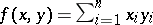, respectively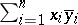.